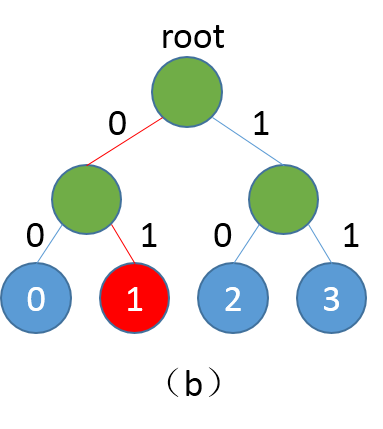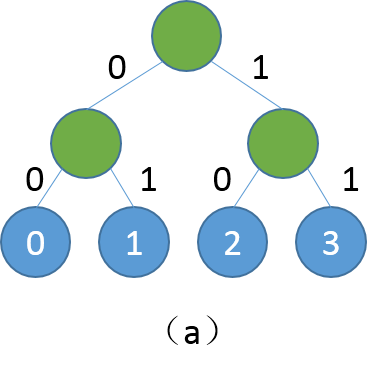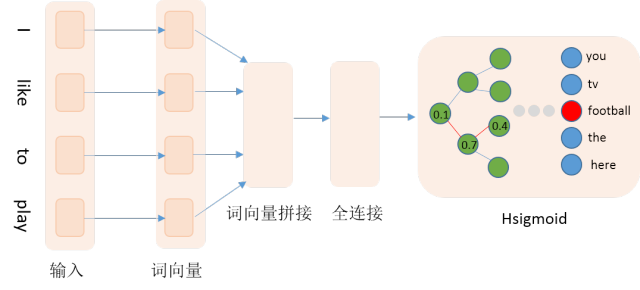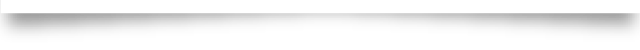# 【词向量】Hsigmoid加速词向量训练

2018/02/28 17:15Hsigmoid加速词向量训练|1.背景介绍

|2. Hsigmoid Layer

Hsigmoid Layer引用自论文，Hsigmoid指Hierarchical-sigmoid，原理是通过构建一个分类二叉树来降低计算复杂度，二叉树中每个叶子节点代表一个类别，每个非叶子节点代表一个二类别分类器。例如我们一共有4个类别分别是0、1、2、3，softmax会分别计算4个类别的得分，然后归一化得到概率。当类别数很多时，计算每个类别的概率非常耗时，Hsigmoid Layer会根据类别数构建一个平衡二叉树，如下：|3. 数据准备

A.PTB数据

B.自定义数据

def reader_creator(filename, word_dict, n):

with open(filename) as f:

UNK = word_dict['<unk>']

for l in f:

l = ['<s>'] + l.strip().split() + ['<e>']

if len(l) >= n:

l = [word_dict.get(w, UNK) for w in l]

for i in range(n, len(l) + 1):

yield tuple(l[i - n:i])

def train_data(filename, word_dict, n):

"""

Reader interface for training data.

It returns a reader creator, each sample in the reader is a word ID tuple.

:param filename: path of data file

:type filename: str

:param word_dict: word dictionary

:type word_dict: dict

:param n: sliding window size

:type n: int

"""

return reader_creator(filename, word_dict, n)

|4.网络结构def ngram_lm(hidden_size, embed_size, dict_size, gram_num=4, is_train=True):

emb_layers = []

name="_proj", initial_std=0.001, learning_rate=1, l2_rate=0)

for i in range(gram_num):

name="__word%02d__" % (i),

emb_layers.append(

input=word, size=embed_size, param_attr=embed_param_attr))

input=embed_context,

size=hidden_size,

initial_std=1. / math.sqrt(embed_size * 8), learning_rate=1))

input=hidden_layer,

label=target_word,

size=dict_size - 1,

|5. 训练阶段

|6. 预测阶段

python infer.py \

--model_path "models/XX" \

--batch_size 1 \

--use_gpu false \

--trainer_count 1

def decode_res(infer_res, dict_size):

"""

Inferring probabilities are orginized as a complete binary tree.

The actual labels are leaves (indices are counted from class number).

This function travels paths decoded from inferring results.

If the probability >0.5 then go to right child, otherwise go to left child.

param infer_res: inferring result

param dict_size: class number

return predict_lbls: actual class

"""

predict_lbls = []

infer_res = infer_res > 0.5

for i, probs in enumerate(infer_res):

idx = 0

result = 1

while idx < len(probs):

result <<= 1

if probs[idx]:

result |=1

if probs[idx]:

idx = idx * 2 + 2  # right child

else:

idx = idx * 2 + 1  # left child

predict_lbl = result - dict_size

predict_lbls.append(predict_lbl)

return predict_lbls

【参考文献】

1. Morin, F., & Bengio, Y. (2005, January). Hierarchical Probabilistic Neural Network Language Model. In Aistats (Vol. 5, pp. 246-252)

end

*原创贴，版权所有，未经许可，禁止转载

*欢迎在留言区分享您的观点### 作者的其它热门文章

0
0 收藏

0 评论
0 收藏
0